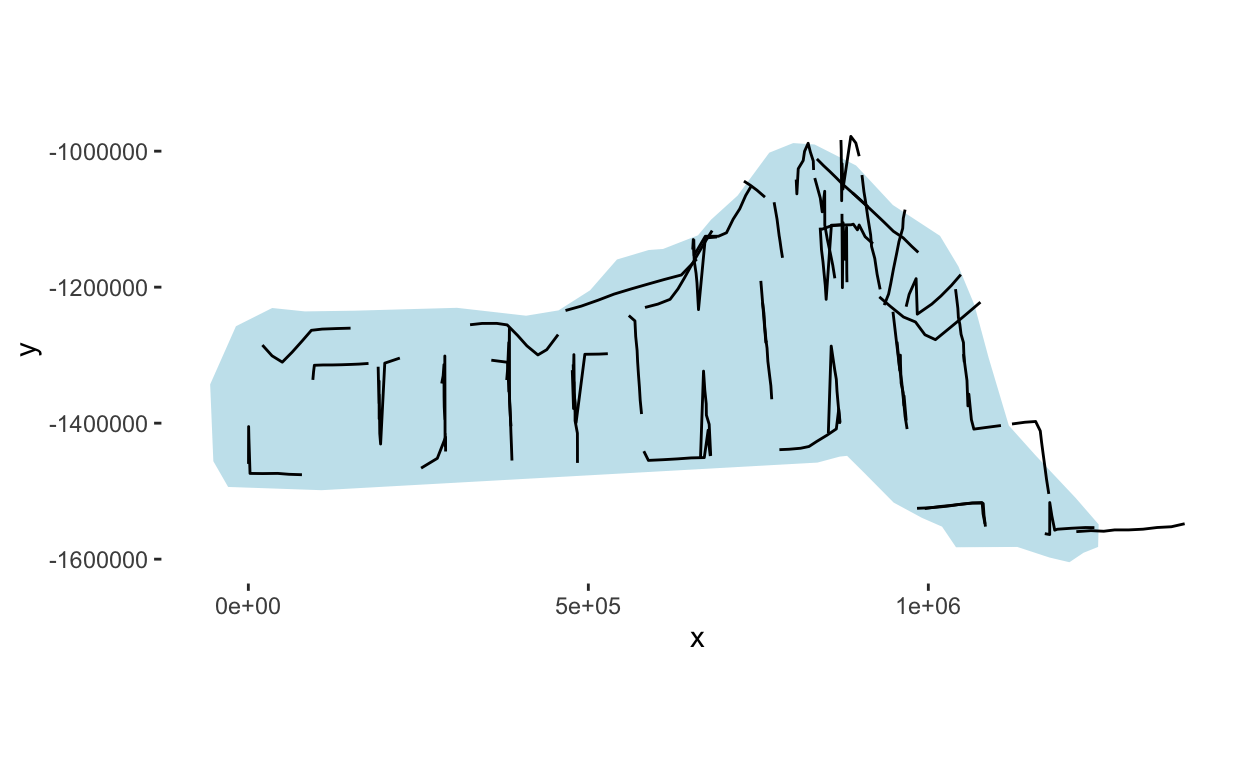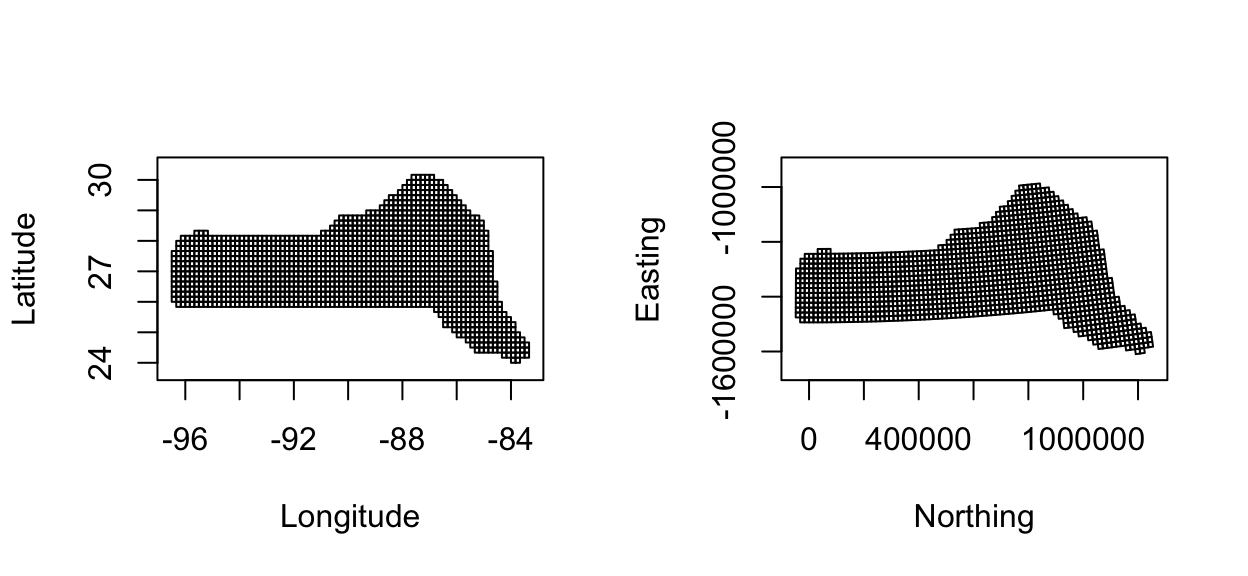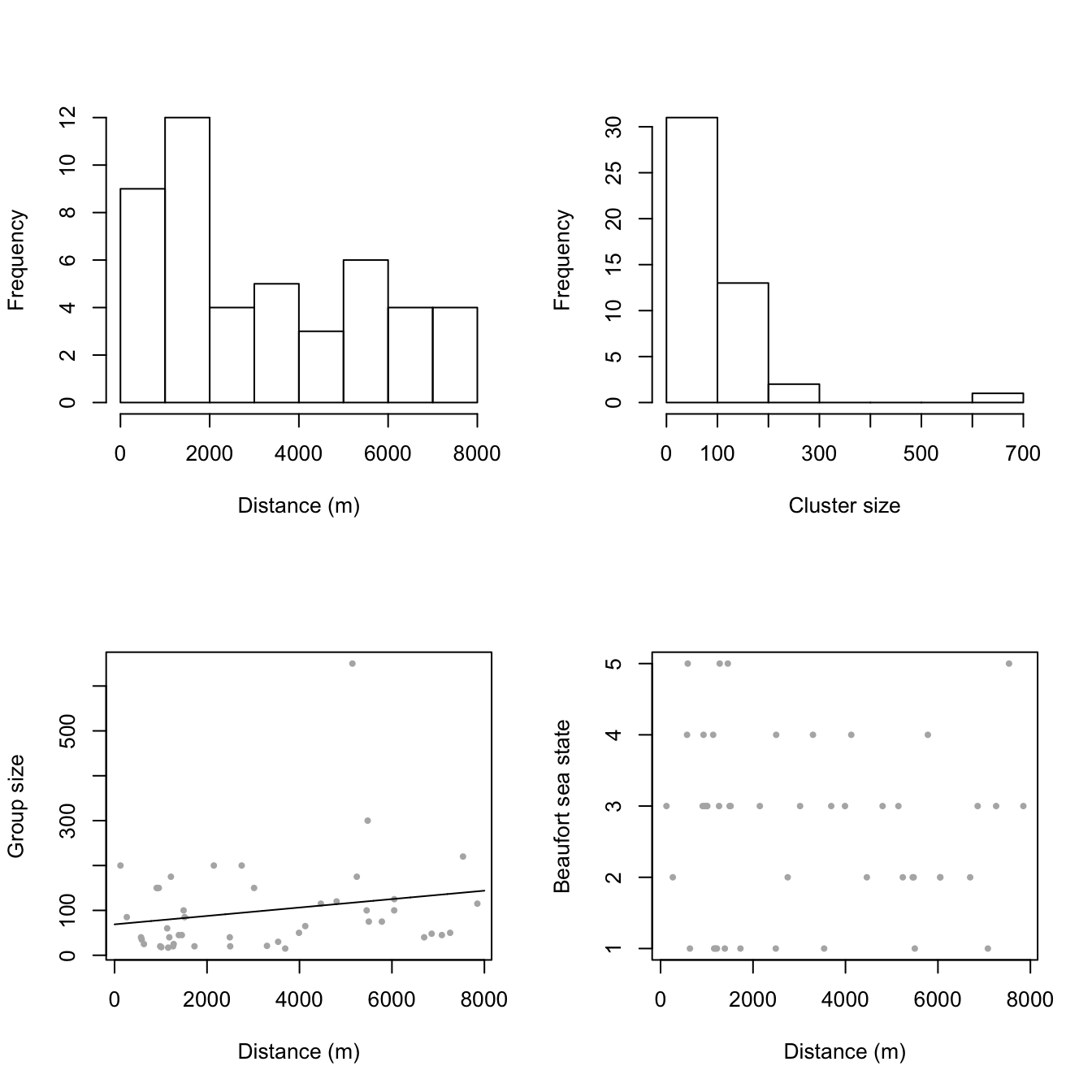# Example dsm analysis: pantropical dolphins in the Gulf of Mexico

Example spatial analysis of pantropical spotted dolphins.

David L Miller http://distancesampling.org (CREEM, Univ of St Andrews)https://creem.st-andrews.ac.uk
2020-04-21

# Introduction

The analysis is based on a dataset of observations of pantropical dolphins in the Gulf of Mexico (shipped with Distance 6.0 and later). For convenience the data are bundled in an R-friendly format, although all of the code necessary for creating the data from the Distance project files is available on github. The OBIS-SEAMAP page for the data may be found at the SEFSC GoMex Oceanic 1996 survey page.

The intention here is to highlight the features of the dsm package, rather than perform a full analysis of the data. For that reason, some important steps are not fully explored. Some familiarity with density surface modelling (Miller, Burt, Rexstad, & Thomas, 2013) (Hedley & Buckland, 2004) is assumed.

# Preamble

Before we start, we load the dsm package (and its dependencies) and set some options:


library(dsm)
library(ggplot2)

# plotting options
gg.opts <- theme(panel.grid.major=element_blank(),
panel.grid.minor=element_blank(),
panel.background=element_blank())

In order to run this vignette, you’ll need to install a few R packages. This can be done via the following call to install.packages:


install.packages(c("dsm", "Distance", "knitr", "distill", "ggplot2", "rgdal",
"maptools", "plyr", "tweedie"))

# The data

Most of the data we need is included in the dsm package, but two additional objects needed for plotting are required and can be downloaded here and should be put into the same directory as this file. The data can then be loaded into R using the following code:


load("mexdolphins-extra.rda")

This should add the objects survey.area and pred.polys to your environment.

## Observation and segment data

All of the data for this analysis has been nicely pre-formatted and is shipped with dsm. Loading that data, we can see that we have four data.frames, the first few lines of each are shown:


data(mexdolphins)

segdata holds the segment data: the transects have already been “chopped” into segments.


head(segdata)

longitude latitude        x        y Effort Transect.Label
1 -86.92712 29.94378 836105.9 -1011416  13800       19960417
2 -86.83176 29.84030 846012.9 -1021407  14000       19960417
3 -86.74445 29.75279 855022.6 -1029785  14000       19960417
4 -86.65230 29.65522 864610.3 -1039168  13900       19960417
5 -86.56648 29.56088 873598.1 -1048266  13800       19960417
6 -86.49290 29.49000 881203.7 -1055004  13800       19960417
Sample.Label depth
1   19960417-1 135.0
2   19960417-2 147.7
3   19960417-3 152.1
4   19960417-4 163.8
5   19960417-5 179.7
6   19960417-6 188.5

distdata holds the distance sampling data that will be used to fit the detection function.


head(distdata)

object size  distance Effort detected beaufort latitude longitude
45      45   21 3296.6363  36300        1        4 27.72872 -86.00159
61      61  150  929.1937  17800        1        4 25.99896 -87.62712
63      63  125 6051.0009  21000        1        2 26.00693 -87.94881
85      85   75 5499.6971  21800        1        1 27.50344 -90.44891
114    114   50 7258.9837  13400        1        3 27.40568 -94.99483
120    120   45 1454.7962  20900        1        5 26.01765 -95.97449
x        y
45  948000.065 -1236192
61  812161.653 -1436899
63  780969.520 -1438985
85  528656.807 -1297833
114  95910.149 -1324562
120   2477.665 -1473909

obsdata links the distance data to the segments.


head(obsdata)

object Sample.Label size  distance Effort
45      45   19960421-9   21 3296.6363  36300
61      61   19960423-7  150  929.1937  17800
63      63   19960423-9  125 6051.0009  21000
85      85   19960427-1   75 5499.6971  21800
114    114   19960430-8   50 7258.9837  13400
120    120   19960501-5   45 1454.7962  20900

preddata holds the prediction grid (which includes all the necessary covariates).


head(preddata)

latitude longitude        x          y depth      area
1 30.08333 -87.58333 774402.9 -1002759.1    35 271236913
2 30.08333 -87.41667 789688.6 -1001264.5    30 271236913
3 30.08333 -87.25000 804971.3  -999740.6    27 271236913
4 30.08333 -87.08333 820251.1  -998187.5    22 271236913
5 30.08333 -86.91667 835528.0  -996605.2    46 271236913
6 29.91667 -87.75000 760783.1 -1021810.3    14 271236913

Typically (i.e. for other datasets) it will be necessary divide the transects into segments, and allocate observations to the correct segments using a GIS or other similar package1, before starting an analysis using dsm.

## Shapefiles and converting units

Often data in a spatial analysis comes from many different sources. It is important to ensure that the measurements to be used in the analysis are in compatible units, otherwise the resulting estimates will be incorrect or hard to interpret. Having all of our measurements in SI units from the outset removes the need for conversion later, making life much easier.

The data are already in the appropriate units (Northings and Eastings: kilometres from a centroid, projected using the North American Lambert Conformal Conic projection).

There is extensive literature about when particular projections of latitude and longitude are appropriate and we highly recommend the reader review this for their particular study area; (Bivand, Pebesma, & Gómez-Rubio, 2008) is a good starting point. The other data frames have already had their measurements appropriately converted. By convention the directions are named x and y.

Using latitude and longitude when performing spatial smoothing can be problematic when certain smoother bases are used. In particular when bivariate isotropic bases are used the non-isotropic nature of latitude and longitude is inconsistent (moving one degree in one direction is not the same as moving one degree in the other).

We give an example of projecting the polygon that defines the survey area (which as simply been read into R using readShapeSpatial from a shapefile produced by GIS).


library(rgdal)
library(maptools)
library(plyr)

# tell R that the survey.area object is currently in lat/long
proj4string(survey.area) <- CRS("+proj=longlat +datum=WGS84")

# proj 4 string
# using http://spatialreference.org/ref/esri/north-america-lambert-conformal-conic/
lcc_proj4 <- CRS("+proj=lcc +lat_1=20 +lat_2=60 +lat_0=40 +lon_0=-96 +x_0=0 +y_0=0 +ellps=GRS80 +datum=NAD83 +units=m +no_defs ")

# project using LCC
survey.area <- spTransform(survey.area, CRSobj=lcc_proj4)

# simplify the object
survey.area <- data.frame(survey.area@polygons[]@Polygons[]@coords)
names(survey.area) <- c("x", "y")

The below code generates this plot, which shows the survey area with the transect lines overlaid (using data from segdata).


p <- qplot(data=survey.area, x=x, y=y, geom="polygon",fill=I("lightblue"),
ylab="y", xlab="x", alpha=I(0.7))
p <- p + coord_equal()
p <- p + geom_line(aes(x,y,group=Transect.Label),data=segdata)
p <- p + gg.opts

print(p)Also note that since we’ve projected our prediction grid, the “squares” don’t look quite like squares. So for plotting we’ll use the polygons that we’ve saved, these polygons (stored in pred.polys) are read from a shapefile created in GIS, the object itself is of class SpatialPolygons from the sp package. This plotting method makes plotting take a little longer, but avoids gaps and overplotting. The following plot compares using latitude/longitude (left) with a projection (right).


par(mfrow=c(1,2))

# put pred.polys into lat/long
pred_latlong <- spTransform(pred.polys,CRSobj=CRS("+proj=longlat +datum=WGS84"))

# plot latlong
plot(pred_latlong, xlab="Longitude", ylab="Latitude")
axis(1); axis(2); box()

# plot as projected
plot(pred.polys, xlab="Northing", ylab="Easting")
axis(1); axis(2); box()Tips on plotting polygons are available from the ggplot2 wiki.

Here we define a convenience function to generate an appropriate data structure for ggplot2 to plot:


# given the argument fill (the covariate vector to use as the fill) and a name,
# return a geom_polygon object
# fill must be in the same order as the polygon data
grid_plot_obj <- function(fill, name, sp){

# what was the data supplied?
names(fill) <- NULL
row.names(fill) <- NULL
data <- data.frame(fill)
names(data) <- name

spdf <- SpatialPolygonsDataFrame(sp, data)
spdf@data$id <- rownames(spdf@data) spdf.points <- fortify(spdf, region="id") spdf.df <- join(spdf.points, spdf@data, by="id") # seems to store the x/y even when projected as labelled as # "long" and "lat" spdf.df$x <- spdf.df$long spdf.df$y <- spdf.df$lat geom_polygon(aes_string(x="x",y="y",fill=name, group="group"), data=spdf.df) } # Exploratory data analysis ## Distance data The top panels of the EDA plots below show histograms of observed distances and cluster size, while the bottom panels show the relationship between observed distance and observed cluster size, and the relationship between observed distance and Beaufort sea state. The plots show that there is some relationship between cluster size and observed distance (fewer smaller clusters seem to be seen at larger distances). The following code generates the EDA plots:  par(mfrow=c(2,2)) # histograms hist(distdata$distance,main="",xlab="Distance (m)")
hist(distdata$size,main="",xlab="Cluster size") # plots of distance vs. cluster size plot(distdata$distance, distdata$size, main="", xlab="Distance (m)", ylab="Group size", pch=19, cex=0.5, col=gray(0.7)) # lm fit l.dat <- data.frame(distance=seq(0,8000,len=1000)) lo <- lm(size~distance, data=distdata) lines(l.dat$distance, as.vector(predict(lo,l.dat)))

plot(distdata$distance,distdata$beaufort, main="", xlab="Distance (m)",
ylab="Beaufort sea state", pch=19, cex=0.5, col=gray(0.7))Top row, left to right: histograms of distance and cluster size; bottom row: plot of distance against cluster size and plot of distances against Beaufort sea state.

## Spatial data

Looking separately at the spatial data without thinking about the distances, we can plot the observed group sizes in space (point size is proportional to the group size for each observation). Circle size indicates the size of the group in the observation. There are rather large areas with no observations, which might cause our variance estimates for abundance to be rather large. We also see the depth data which we will use depth later as an explanatory covariate in our spatial model.


p <- ggplot() + grid_plot_obj(preddata\$depth, "Depth", pred.polys) + coord_equal()
p <- p + labs(fill="Depth",x="x",y="y",size="Group size")
p <- p + geom_line(aes(x, y, group=Transect.Label), data=segdata)
p <- p + geom_point(aes(x, y, size=size), data=distdata, colour="red",alpha=I(0.7))
p <- p + gg.opts
print(p)